MAS.020UF Introduction to Solid State Physics

## Crystal structure

In a crystal, atoms are arranged in straight rows in a three-dimensional periodic pattern. A small part of the crystal that can be repeated to form the entire crystal is called a unit cell.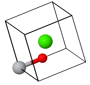Asymmetric unitPrimitive unit cell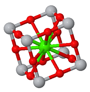Conventional unit cell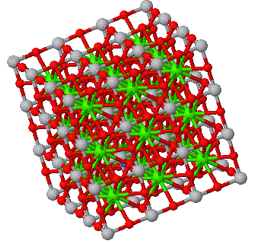Crystal

Devices such as solid state transistors, lasers, solar cells, and light emitting diodes are often made from single crystals. Many materials, including most metals and ceramics, are polycrystaline. This means there are many little crystals packed together where the orientation between the crystals is random. When the atoms of a material are not arranged in a regular pattern, it is called an amorphous material. An example of an amorphous material is window glass. Even though not all solids are crystals, we will spend most of our time studying crystals since the translational symmetry makes them easier to decribe mathematically. Describing the behavior of more complicating materials usually builds on the understanding that has been acquired by studying crystals.

Some common crystal structures you should know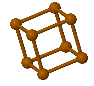Simple Cubic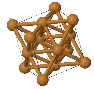Face Centered Cubic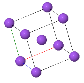Body Centered Cubic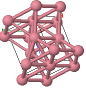Hexagonal Close Packed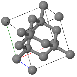Diamond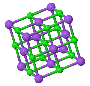NaCl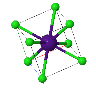CsCl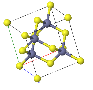ZincblendeWurzite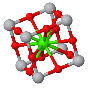Perovskite

Kittel Chapter 1: Crystal Structure or R. Gross und A. Marx: Kristallstrucktur 1.1 - 1.2

For the exam you should
• know that a crystal consists of a basis (the atoms of a primitive unit cell) and one of the 14 Bravais lattices. You should be able to draw the conventional unit cell given the basis and the Bravais lattice as in this problem.
• know what the primitive lattice vectors $(\vec{a}_1,\vec{a}_2,\vec{a}_3)$ are and how they can be used to calculate the volume of a primitive unit cell.
• The volume of a unit cell is $\vec{a}_1\cdot(\vec{a}_2\times\vec{a}_3)$.
• A translation vector of the crystal is $\vec{T}=l\vec{a}_1+m\vec{a}_2+n\vec{a}_3$ where $l,m,n$ are integers.
• be able to draw the following crystal structures: simple cubic, fcc, bcc, hcp, NaCl, CsCl, hexagonal, tetragonal, and orthorhombic.
• be able to construct a Wigner Seitz cell. This is a primitive unit cell with the same symmetry as the crystal.
• know how Miller indicies are used to define directions and planes in a crystal. You should be able to draw the arrangement of atoms at the surface of a crystal cut along a plane specified by Miller indices such as in this problem.
• know what an asymmetric unit is and how it can be used to specify a crystal structure.
• Every crystal structure has a set of symmetries associated with it. These are specified by the point group, the space group, and the Bravais lattice. The space group uniquely determines the point group and the Bravais lattice.
• Every crystal can be associated with one of the 32 point groups. A point group is the collection of symmetries (rotations, reflections, inversion) that a crystal has where at least one point in the crystal does not move during the transformation. Point group symmetries can be represented by $3\times 3$ matrices. Some examples are:
Rotation about the $x$-axis by
Rotation about the $y$-axis by
Rotation about the $z$-axis by
Rotoinversion about the $x$-axis by an angle
Rotoinversion about the $y$-axis by an angle
Rotoinversion about the $z$-axis by an angle
$$\text{Identity }E= \left[\begin{matrix} 1 & 0 & 0 \\ 0 & 1 & 0 \\ 0 & 0 & 1 \end{matrix}\right]$$